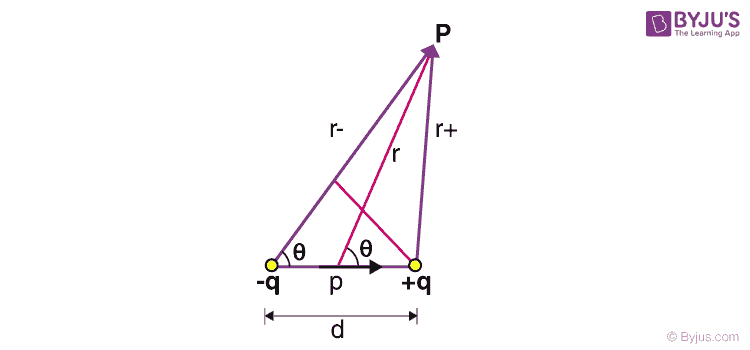# Electric Potential of a Dipole and System of Charges

Before we understand the characteristics of the electric potential of a dipole, let us quickly review our understanding of dipole and electric potential. An electric potential is the amount of work needed to move a unit positive charge from a reference point to a specific point inside an electric field without producing acceleration. A dipole is a pair of opposite charges with equal magnitudes separated by a distance, d.

The electric potential due to a point charge q at a distance of r from that charge is given by,

$$\begin{array}{l}V = \frac{1}{4πε_0}~\frac{q}{r}\end{array}$$
Where ε0 is the permittivity of free space.

The electric potential is a scalar field whose gradient becomes the electrostatic vector field. Since it is a scalar field, it is easy to find the potential due to a system of charges. It is the summation of the electric potentials at a point due to individual charges.

Thus, we can write the net electric potential due to the individual potentials contributed by charges as

$$\begin{array}{l}V_{net} = \sum\limits_{i}~V_{i}\end{array}$$

$$\begin{array}{l}V_{net} = \frac{1}{4πε_0}~\sum\limits_{i}~\frac{q_i}{r_i}\end{array}$$

Let us use this concept to find the electric field of a dipole. Let the distance between the point P and the positive and negative charges be r+ and r respectively. Let the distance from the midpoint of the dipole be r. Let this vector subtend an angle θ to the dipole axis. Thus using the above theorem, we have,

$$\begin{array}{l}V = \sum_{i}~V_i = V_{+}~+~V_{-}\end{array}$$
$$\begin{array}{l}V = \frac{1}{4πε_0}~\left(\frac{q}{r_{+}}~ +~\frac{-q}{r_{-}}\right)\end{array}$$$$\begin{array}{l}V = \frac{q}{4πε_0}\left(\frac{1}{r_{+}}~ -~\frac{1}{r_{-}} \right)\end{array}$$
$$\begin{array}{l}V = \frac{q}{4πε_0}~\left(\frac{r_{-}~-~r_{+}}{r_{+}~ r_{-}}\right)\end{array}$$

If the point P is sufficiently far from the dipole, then we can approximate

$$\begin{array}{l}r_{-} ≈ r_{+} ≈ r\end{array}$$
And by drawing a line perpendicular to r from +q we can write,
$$\begin{array}{l}r_{-}~-~r_{+} ≈ d cos~θ\end{array}$$

Thus, we can write the potential as:

$$\begin{array}{l}V = \frac{q}{4πε_0}~\left(\frac{d ~cos~θ}{r^2}\right)\end{array}$$

We know that the magnitude of the electric dipole moment is:

$$\begin{array}{l}|\overrightarrow{p}| = p = q~.~d\end{array}$$

Thus, electric potential due to a dipole at a point far away from the dipole is given by,

$$\begin{array}{l}V = \frac{1}{4πε_0}~ \frac{p~ cos~θ}{r^2}\end{array}$$

Notice that the potential falls off by r2 while the electric field falls off by r3. Also, note that when the angle is 90⁰, the point P is equidistant to both charges, and the electric potential is zero. When θ > 90⁰, the potential is negative because the point P is closer to the negative charge.

## Important JEE questions on Electrostatic Potential and Capacitance## Know About Electric Field due to Dipoles

## Frequently Asked Questions – FAQs

Q1

### What is an electric charge?

Electric charge is the basic physical property of matter that causes it to experience a force when kept in an electric or magnetic field. An electric charge is associated with an electric field, and the moving electric charge generates a magnetic field. An electric charge can be negative or positive. Unlike charges attract and like charges repel each other.
Q2

### What is electric potential?

Electric potential is the amount of work required to displace a unit charge from a reference point to the desired point against an electric field.
Q3

### What is the SI unit of the dipole moment?

The SI unit of dipole moment is coulomb metre.
Q4

### What is an electric dipole?

An electric dipole is defined as a couple of opposite charges q and –q separated by a distance d. The midpoint q and –q is called the centre of dipole.
Q5

### What is the direction of an electric dipole moment?

The electric dipole moment is a vector quantity, and it has a well-defined direction which is from the negative charge to the positive charge.

Stay tuned with BYJU’S for more such interesting articles. Also, register to “BYJU’S – The Learning App” for loads of interactive, engaging Physics-related videos and unlimited academic assistance.

Test Your Knowledge On Electric Potential Dipole!